# Derivation Applications of Bernoulli PrincipalPage 1

#### WATCH ALL SLIDES

Slide 1## Lesson Opener

How does a plane fly?

How does a perfume spray work?

Why does a cricket ball curve?

Slide 2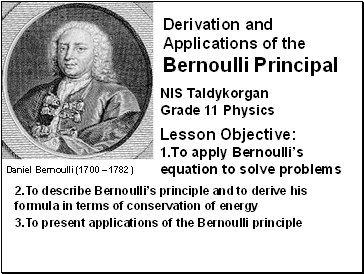## Daniel Bernoulli (1700 – 1782)

Derivation and Applications of the Bernoulli Principal

NIS Taldykorgan

Lesson Objective:

1.To apply Bernoulli’s equation to solve problems

2.To describe Bernoulli’s principle and to derive his formula in terms of conservation of energy

3.To present applications of the Bernoulli principle

Slide 3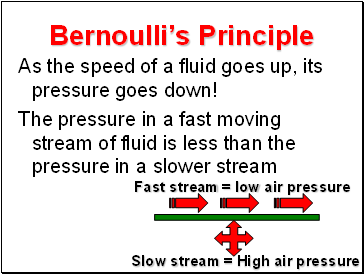## Bernoulli’s Principle

As the speed of a fluid goes up, its pressure goes down!

The pressure in a fast moving stream of fluid is less than the pressure in a slower stream

Fast stream = low air pressure

Slow stream = High air pressure

Slide 4Slide 5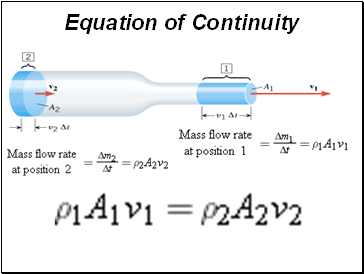## Equation of Continuity

Slide 6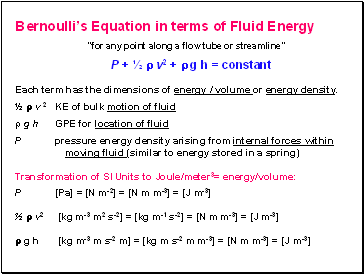## Bernoulli’s Equation in terms of Fluid Energy

“for any point along a flow tube or streamline”

P + ½  v2 +  g h = constant

Each term has the dimensions of energy / volume or energy density.

½  v 2 KE of bulk motion of fluid

g h GPE for location of fluid

P pressure energy density arising from internal forces within

moving fluid (similar to energy stored in a spring)

Transformation of SI Units to Joule/meter3= energy/volume:

P [Pa] = [N m-2] = [N m m-3] = [J m-3]

½  v2 [kg m-3 m2 s-2] = [kg m-1 s-2] = [N m m-3] = [J m-3]

 g h [kg m-3 m s-2 m] = [kg m s-2 m m-3] = [N m m-3] = [J m-3]

Slide 7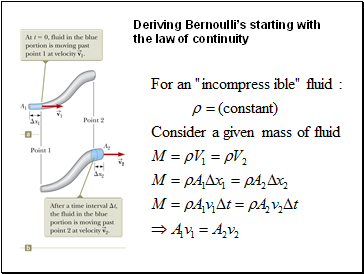## Deriving Bernoulli’s starting with the law of continuity

Slide 8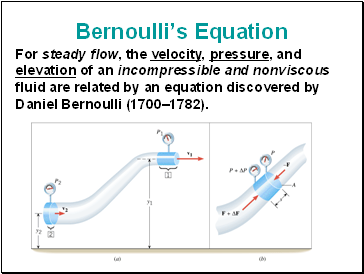## Bernoulli’s Equation

For steady flow, the velocity, pressure, and elevation of an incompressible and nonviscous fluid are related by an equation discovered by Daniel Bernoulli (1700–1782).

Slide 9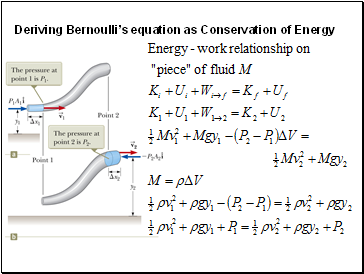Deriving Bernoulli’s equation as Conservation of Energy

Slide 10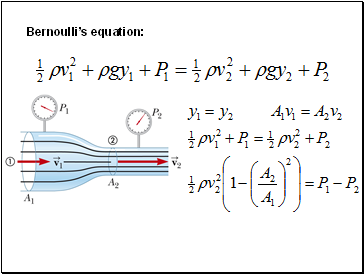Bernoulli’s equation:

Slide 11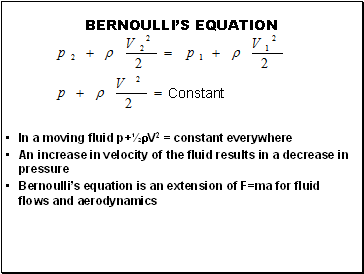BERNOULLI’S EQUATION

In a moving fluid p+½rV2 = constant everywhere

Go to page:
1  2